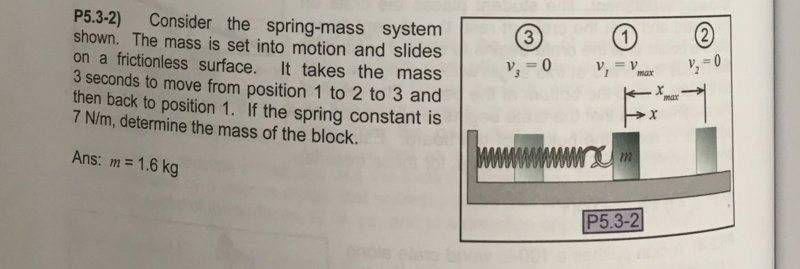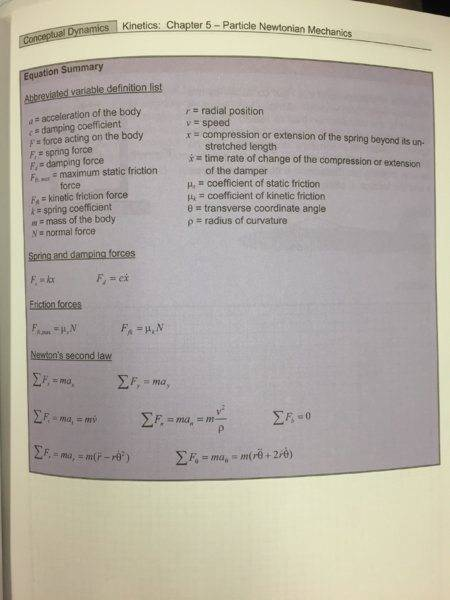# Finding the mass of a block attached to a spring

## Homework Statement

What is the mass of the block?

Given: friction less surface, velocity at each marked point, distance between points 1 and 2, spring constant l = 7 N/m

## Homework Equations

F=ma
Kinematic equations

## The Attempt at a Solution

I tried to solve for a in m = F/a in order to find the mass. My units don't seem to agree, which suggests I have done something wrong.

#### Attachments

gneill
Mentor

Edit: Also a hint: What's the formula for the period of a spring-mass system?

SammyS
Staff Emeritus
Homework Helper
Gold Member

## Homework Statement

What is the mass of the block?

Given: friction less surface, velocity at each marked point, distance between points 1 and 2, spring constant l = 7 N/m
No, you are not given any distance nor any velocity.## Homework Equations

F=ma
Kinematic equations

## The Attempt at a Solution

I tried to solve for a in m = F/a in order to find the mass. My units don't seem to agree, which suggests I have done something wrong.
Yes, from the little I make out in the image of your solution, you are trying to solve this using the kinematic equations for constant acceleration. That's not the case here at all.

#### Attachments

Edit: Also a hint: What's the formula for the period of a spring-mass system?
Can't be using formulas for periods, haven't technically learned that yet. Must use other methods. Sorry about the image

No, you are not given any distance nor any velocity.
View attachment 232766

Yes, from the little I make out in the image of your solution, you are trying to solve this using the kinematic equations for constant acceleration. That's not the case here at all.
Any hints on how to find acceleration then? I can only use force methods. No energy, harmonic stuff, or periods

SammyS
Staff Emeritus
Homework Helper
Gold Member
Any hints on how to find acceleration then? I can only use force methods. No energy, harmonic stuff, or periods
Can you solve differential equations?

Also, unless you post something more readable, you are not likely to get many replies.

Can you solve differential equations?
Uh no unfortunately

Then it seems you are expected to know the formula for the period of a mass-spring system.
Try http://hyperphysics.phy-astr.gsu.edu/hbase/shm2.html
Yes, but this was unfortunately not covered in the section from which this problem was taken. I'm trying to see how to solve without using this.

gneill
Mentor
Yes, but this was unfortunately not covered in the section from which this problem was taken. I'm trying to see how to solve without using this.
Yikes! I recall that this was one of the "given" formulas that I was presented with in high-school physics (without proof at that time) to use when faced with these sorts of problems.

Can you list the equations related to this type of problem that you've been given?

gneill
Mentor
Yeah, that image just about impossible to read. But I don't see anything recognizable as being specifically related to harmonic motion. So that's going to pose a significant problem if you haven't done differential equations. Since the problem statement specifically points out the period of the harmonic motion, it seems strange that you haven't been introduced to the equation for the period of a spring-mass oscillator.

SammyS
Staff Emeritus
Homework Helper
Gold Member
Yes, but this was unfortunately not covered in the section from which this problem was taken. I'm trying to see how to solve without using this.
What topics / equations are covered in this section of the book ?

What topics / equations are covered in this section of the book ?
Literally just F=ma in various forms (normal tangential, polar) and a damping force was mentioned F = c * (dx/dt). also F = kx.

gneill
Mentor
I find it hard to fathom that you've been exposed to the concept of damping yet haven't been taught the formula for the fundamental period of a spring-mass system. If I may ask, what course (in your school) does this question come from?

I find it hard to fathom that you've been exposed to the concept of damping yet haven't been taught the formula for the fundamental period of a spring-mass system. If I may ask, what course (in your school) does this question come from?
intro dynamics

gneill
Mentor
intro dynamics
This is a college course? If so, I presume that you'd be able to "access" any methods or formulas that you might have covered in your high-school physics.

I suggest you save yourself a lot of time and fire up Google with "mass spring period".

This is a college course? If so, I presume that you'd be able to "access" any methods or formulas that you might have covered in your high-school physics.

I suggest you save yourself a lot of time and fire up Google with "mass spring period".
We're going to cover those things you mentioned, but later in the course. For now, we're supposed to be using just force methods. The book is known to have a lot of mistakes; it's possible that it could be impossible with just force methods. Do you think that's the case here?

gneill
Mentor
We're going to cover those things you mentioned, but later in the course. For now, we're supposed to be using just force methods. The book is known to have a lot of mistakes; it's possible that it could be impossible with just force methods. Do you think that's the case here?
Then you'll need to write and solve the differential equation.

Then you'll need to write and solve the differential equation.
Can you elaborate a little more? I'm not familiar with differential equations

gneill
Mentor
Can you elaborate a little more? I'm not familiar with differential equations
A differential equation is one that uses differential calculus to relate rates of change of variables with respect to each other. Typically, for example, one might write Newton's second law for a given system using the differential form. Then that equation would be solved using various methods depending upon the specific nature of the equation.

Google "differential equation" to see more/better descriptions and examples.

A differential equation is one that uses differential calculus to relate rates of change of variables with respect to each other. Typically, for example, one might write Newton's second law for a given system using the differential form. Then that equation would be solved using various methods depending upon the specific nature of the equation.

Google "differential equation" to see more/better descriptions and examples.
Ok thanks for the help. I'll use F=ma then and see where I can go from there

gneill
Mentor
Ok thanks for the help. I'll use F=ma then and see where I can go from there
You won't get far without casting it as a differential equation.

SammyS
Staff Emeritus
Homework Helper
Gold Member
(Your image in the attached file:Literally just F=ma in various forms (normal tangential, polar) and a damping force was mentioned F = c * (dx/dt). also F = kx.
Here's an idea:

See if you can relate the motion of the mass attached to the spring, to the x or y component of the motion of a mass undergoing uniform circular motion where the centripetal force is ##\ k\cdot x_\text{max}\,,\ ## the radius of the circle is ##\ x_\text{max}\,,\ ## and the mass travels the complete circle every 3 seconds.

In order for this to be reasonable, the x or y, component (whichever you use) of the centripetal force should match the spring force at corresponding positions.